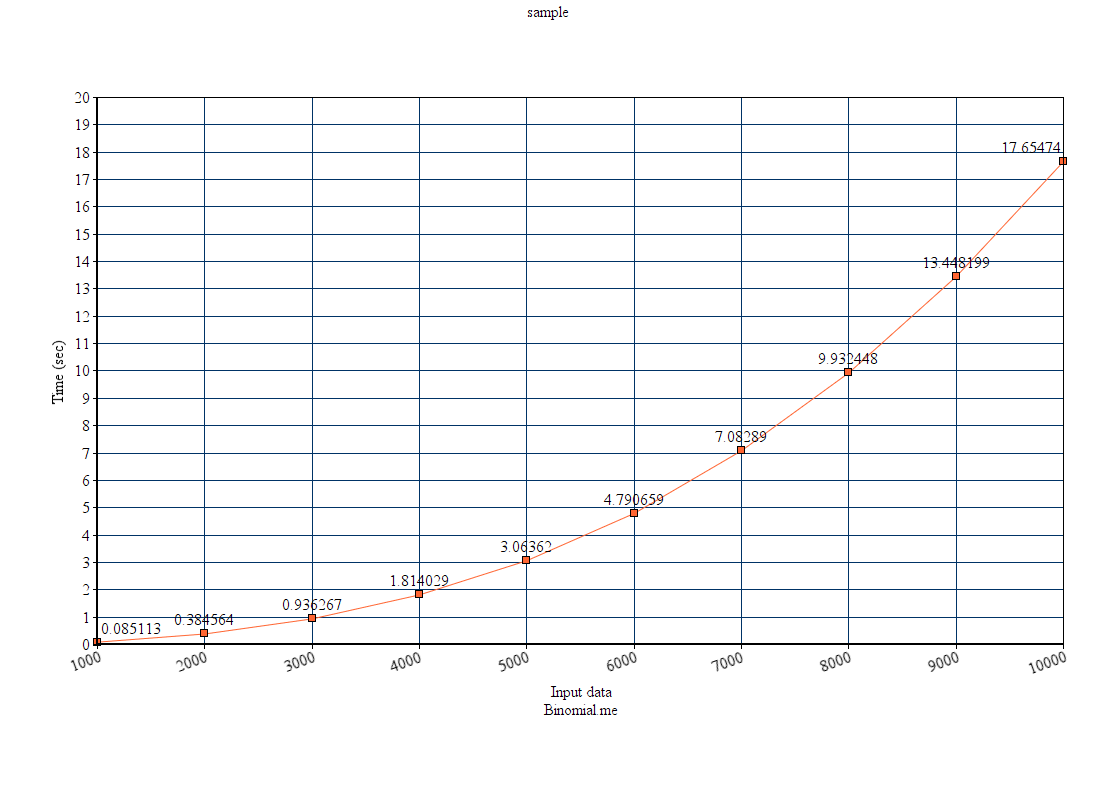Play with a random code

Before I tell what is dp? I would like you to go through an example code.

Some random code:

def F(n):
if n == 0:
return 0
else:
result = 0
for i in range(n):
result += F(i)
return result+n

print F(int(raw_input()))

If you observe the function F(n) is calculating (2**n)-1 with a time complexity of O(2^n). It can be done in O(log2(n)) also, but that’s not the concern here.

The above code is an example of Exhaustive Search where we are exploring every possible branch that can be formed from a set of whole numbers up to n (which is passed as the argument and for loop is used for that). Let’s construct the decision tree (recursive call stack) for the above function.

Let a call be made with F(3), now three branches will be formed for each number in the set S (S is set of whole numbers up to n). I have taken n = 3, coz it will be easy for me to make the diagram for it. You can try will other larger numbers and observe the recursion call stack.

3
/| \
0 1  2    ----> the leftmost node is returns 0 coz (n==0) it's the base case
|  /\
0  0 1
|
0   ----> returns 0

So here you have explored every possibility branches. If you try to write the recursive equation for the above problem then:

T(n) = 1; n is 0
= T(n-1) + T(n-2) + T(n-3) + ... + T(1); otherwise

Here,

T(n-1) = T(n-2) + T(n-3) + ... T(1).
So, T(n-1) + T(n-2) + T(n-3) + ... + T(1) = T(n-1) + T(n-1)

So, the Recursive equation becomes:

T(n) = 1; n is 0
= 2*T(n-1); otherwise

Now you can easily solve this recurrence relation (or use can use Masters theorem for the fast solution). You will get the time complexity as O(2^n).

Solving the recurrence relation:

T(n) = 2T(n-1)
= 2(2T(n-1-1) = 4T(n-2)
= 4(2T(n-3)
= 8T(n-3)
= 2^k T(n-k), for some integer `k` ----> equation 1

Now we are given the base case where n is 0, so let,

n-k = 0 , i.e. k = n;

Put k = n in equation 1,

T(n) = 2^n * T(n-n)
= 2^n * T(0)
= 2^n * 1; // as T(0) is 1
= 2^n

So, T.C = O(2^n)

If you print the recursion call stack on the console you will get something like this:

F(4)
F(0)
F(1)
F(0)
F(2)
F(0)
F(1)
F(0)
F(3)
F(0)
F(1)
F(0)
F(2)
F(0)
F(1)
F(0)
15

Now you can observe how function calls are being made. So you can see the repetation of the computation of F(1) and F(2). Also, if you observe the recursion Tree formed above (each node in the tree is a subproblem of the main problem), you will see that the nodes are repeating (i.e. the subproblems are repeating).

What if we use a memory in our function to store the already computed value and whenever the sub-problems are occurring again (i.e. repeating function calls or repeating nodes) we will use the pre-computed value (this saves time for computing the sub-problems again and again). The approach is also known as Dynamic Programming.

I think the main problem comes in doing the memoization part (i.e. storing the results of expensive function calls and returning the cached result). Again for the what I do is see the decision tree or the recursion call stack (either printed on console or made on notebook) and then figure out where does the repeating nodes (or subproblems) occur. If they occur on same level in the dicision tree then I can just used a simple variable to store the result and that variable can hold the result in that call stack. But if the repeating nodes are occurring on different levels then we might need to use a storage entity like list and pass them on each function calls so that we can use the computed values as an when required.

Now you can scroll up can once again and look at the recursion call stack (or dicision tree), by now you must have figured out that we would need a list as the storage entity to store the pre-computed results (as the overlapping sub-problems are occurring on different levels).

Below is the code which uses dynamic programming approach to optimize the above function.

def F(n, dp):
result = -1
# base case
if n == 0:
dp.append(1)  # putting dp = 1, as its the base case, 2^0=1
return 1
else:
for i in xrange(n):
# check if dp array has already that index value computed or not
if i < len(dp):
result += dp[i]
else:
result += F(i, dp)
dp.append(result+n)
return result+n

if __name__ == '__main__':
n = int(raw_input())
import time
start_time = time.time()
print F(n, [])
print '\n', (time.time()-start_time)

So, that’s how I converted a time consuming recursive program into optimized solution. You should also try to write your solution using dynamic programming approach after understanding the decision tree formed from the recursive solution.

The time complexity of the above function seems to be O(n).

Here I observe that, although that asymptotic time seems to be O(n) but the increase in computation time with respect to the input size doesn’t increase linearly.

Here are some results for program written in python.

Input Computation time(sec)

1000

0.0851130485535

2000

0.384564161301

3000

0.936266899109

4000

1.81402897835

5000

3.06361985207

6000

4.79065895081

7000

7.08289003372

8000

9.93244814873

9000

13.4481990337

10000

17.6547398567

The input vs time graph looks like this: